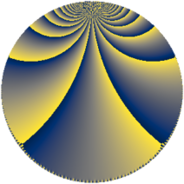# Properties

 Label 304.5.bhLevel $304$ Weight $5$ Character orbit 304.bh Rep. character $\chi_{304}(35,\cdot)$ Character field $\Q(\zeta_{36})$ Dimension $1896$ Sturm bound $200$

# Related objects

## Defining parameters

 Level: $$N$$ $$=$$ $$304 = 2^{4} \cdot 19$$ Weight: $$k$$ $$=$$ $$5$$ Character orbit: $$[\chi]$$ $$=$$ 304.bh (of order $$36$$ and degree $$12$$) Character conductor: $$\operatorname{cond}(\chi)$$ $$=$$ $$304$$ Character field: $$\Q(\zeta_{36})$$ Sturm bound: $$200$$

## Dimensions

The following table gives the dimensions of various subspaces of $$M_{5}(304, [\chi])$$.

Total New Old
Modular forms 1944 1944 0
Cusp forms 1896 1896 0
Eisenstein series 48 48 0

## Trace form

 $$1896q - 12q^{2} - 12q^{3} - 12q^{4} - 12q^{5} - 12q^{6} - 12q^{7} - 6q^{8} + O(q^{10})$$ $$1896q - 12q^{2} - 12q^{3} - 12q^{4} - 12q^{5} - 12q^{6} - 12q^{7} - 6q^{8} + 282q^{10} - 6q^{11} - 6q^{12} - 12q^{13} + 84q^{14} + 1416q^{16} - 24q^{17} - 24q^{18} - 12q^{19} - 24q^{20} + 474q^{21} - 12q^{22} - 24q^{23} - 12q^{24} + 6186q^{26} - 6q^{27} + 1524q^{28} - 12q^{29} - 3240q^{30} - 3162q^{32} - 24q^{33} + 10608q^{34} + 3738q^{35} - 9084q^{36} - 24q^{37} + 618q^{38} - 48q^{39} + 258q^{40} - 27636q^{42} - 12q^{43} + 15630q^{44} - 6q^{45} + 2964q^{46} - 12q^{48} - 300480q^{49} + 16140q^{50} - 12108q^{51} - 18972q^{52} - 12q^{53} - 15606q^{54} - 24q^{55} + 28788q^{56} - 24q^{58} - 12q^{59} + 26742q^{60} - 12q^{61} - 108q^{62} - 6q^{64} - 12q^{65} + 7248q^{66} - 12q^{67} - 19986q^{68} + 29370q^{69} - 31470q^{70} - 24q^{71} - 12576q^{72} + 35016q^{74} - 90072q^{75} - 16380q^{76} + 28788q^{77} - 48804q^{78} - 9552q^{80} - 24q^{81} - 27912q^{82} - 6q^{83} + 28806q^{84} + 67188q^{85} - 12q^{86} - 12q^{87} - 6q^{88} + 83688q^{90} + 14394q^{91} - 12q^{92} - 498q^{93} + 46596q^{94} - 197292q^{96} - 24q^{97} + 29232q^{98} - 39378q^{99} + O(q^{100})$$

## Decomposition of $$S_{5}^{\mathrm{new}}(304, [\chi])$$ into newform subspaces

The newforms in this space have not yet been added to the LMFDB.